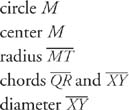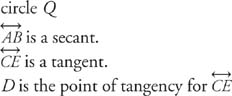## Parts of Circles

A circle is a special figure, and as such has parts with special names. There are also special angles, lines, and line segments that are exclusive to circles. In this chapter, we shall examine all of them.

• Circle: To review, a circle is a planar figure consisting of all the points equidistant from a fixed point.
• Center: That fixed one point is called the center of the circle. Circles are named by naming the center.
• Radius: Any segment with one endpoint at the center of the circle and the other endpoint on the circle is a radius. (The plural of radius is radii.)
• Chord: Any segment whose endpoints lie on the circle is a chord.
• Diameter: Any chord that passes through the center of the circle is a diameter.

In Figure 1:Figure 1 Special points and line segments related to a circle.

From the definition of radius and diameter, it is clear that all radii of a circle are equal in length and all diameters of a circle are equal in length.

• Secant: Any line that contains a chord is a secant.
• Tangent: Any line in the same plane as a circle and intersecting the circle at exactly one point is a tangent.
• Point of tangency: The point where a tangent line intersects a circle is the point of tangency.

In Figure 2:Figure 2 A secant and a tangent to a circle.

• Common tangents: A line that is tangent to two circles in the same plane is a common tangent.
• Internal common tangent: A common tangent that intersects the segment joining the centers of two circles is an internal common tangent.
• External common tangent: A common tangent that does not intersect the segment joining the centers of two circles is an external common tangent.

In Figure 3:

• Lines l and m are common tangents.
• l is an internal common tangent.
• m is an external common tangent.Figure 3 Internal and external common tangents to circles.

Example 1: Use Figure to find each of the following.Figure 4 Identifying special lines and line segments related to circles.

b. chord to circle P

c. diameter of circle O

d. secant to circle P

e. a tangent to circle O

f. a common internal tangent to circles O and P

g. a common external tangent to circles O and P